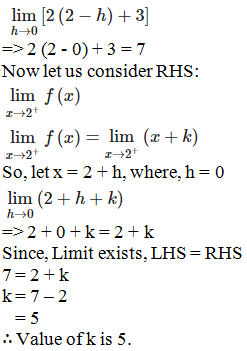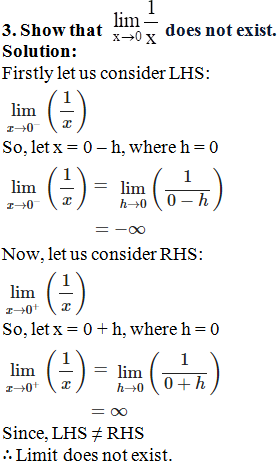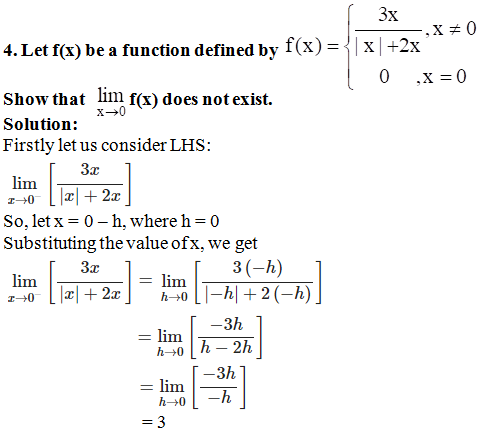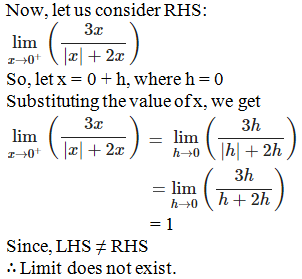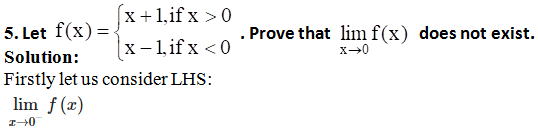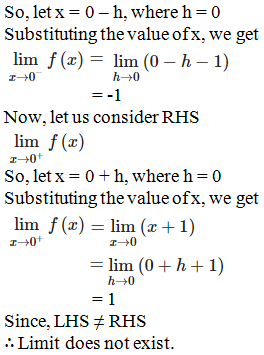# RD Sharma Solutions for Class 11 Chapter 29 - Limits Exercise 29.1

Here, in this exercise, we shall discuss concepts related to informal approach to limit, evaluation of left hand and right hand limits. All questions enlisted in Class 11 Chapter 29 solutions are solved by BYJU’S experts, based on the latest CBSE syllabus and help students gain competence on the concept. From the exam point of view, solutions are designed using shortcut techniques, which helps students to speed up the ability to solve problems. Students may keep the textbook aside and refer to RD Sharma Class 11 Maths Solutions for revision purpose. Students can download the pdf, from the links provided below.

## Download the pdf of RD Sharma Solutions for Class 11 Maths Exercise 29.1 Chapter 29 – Limits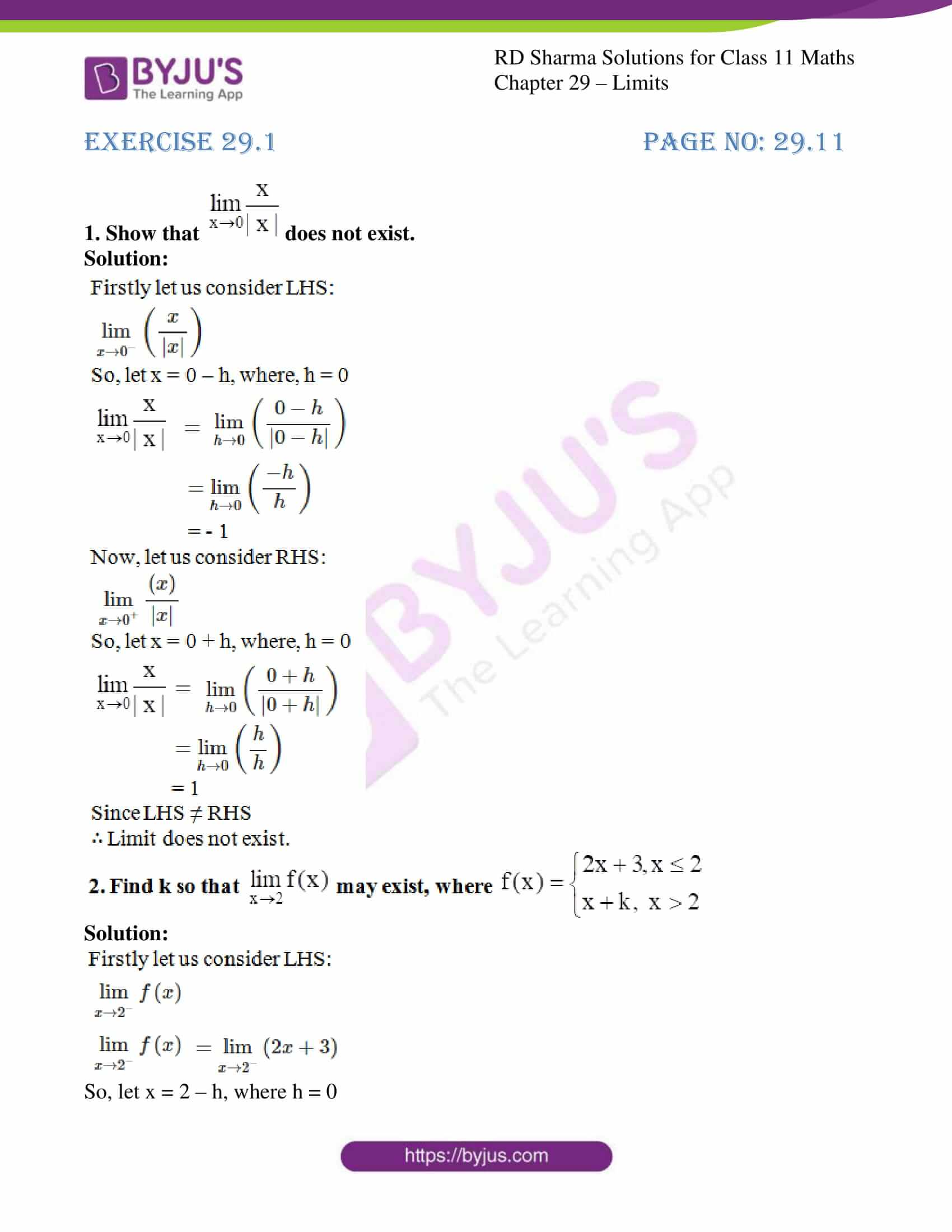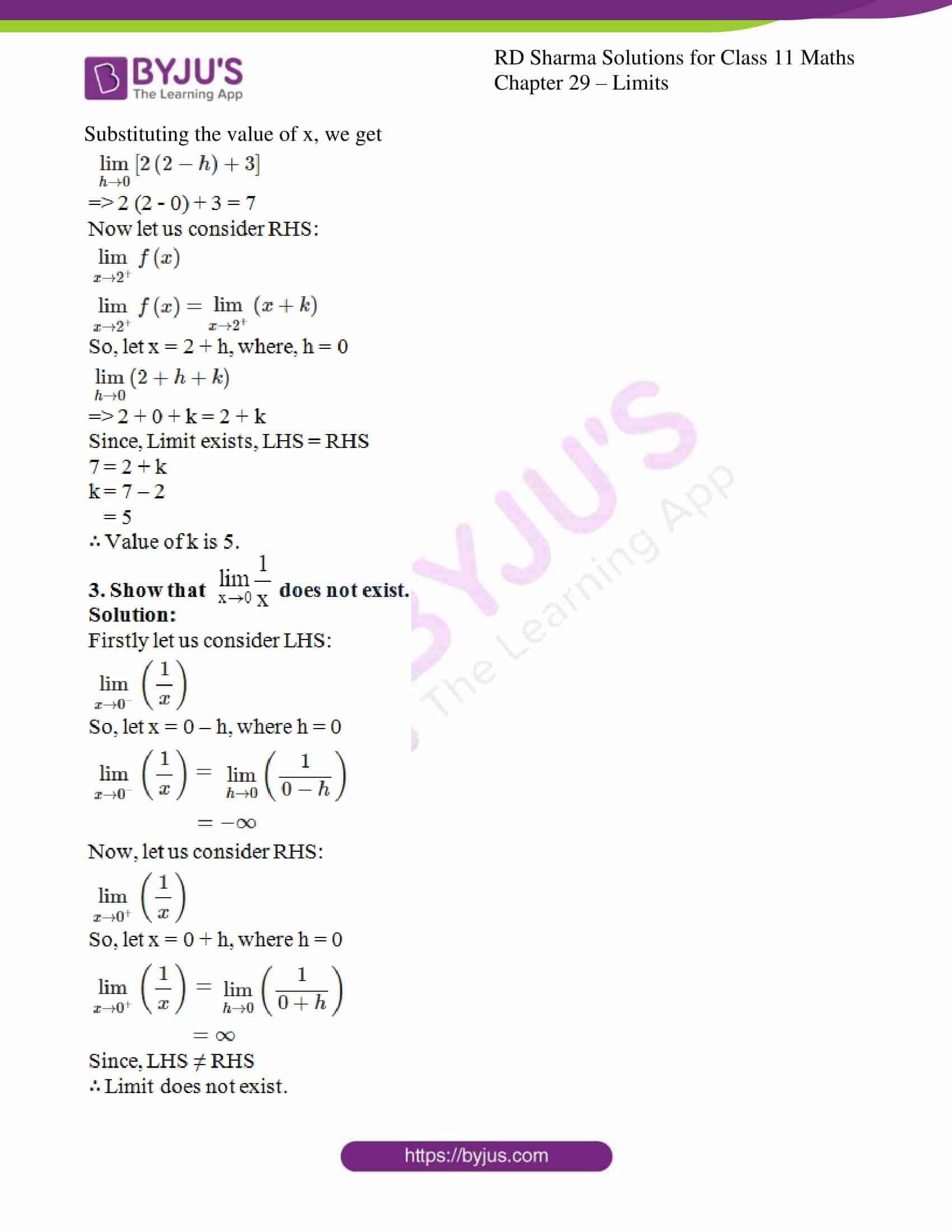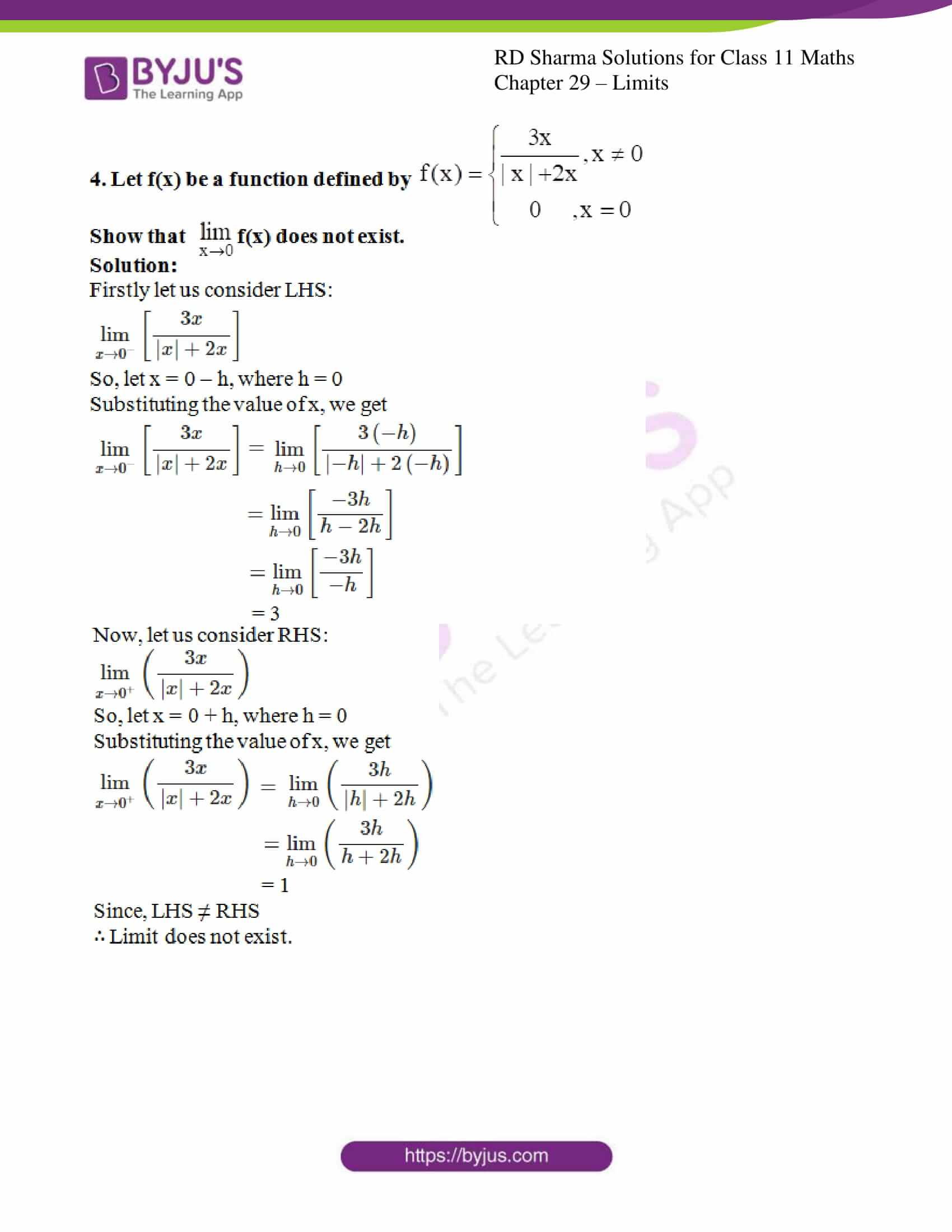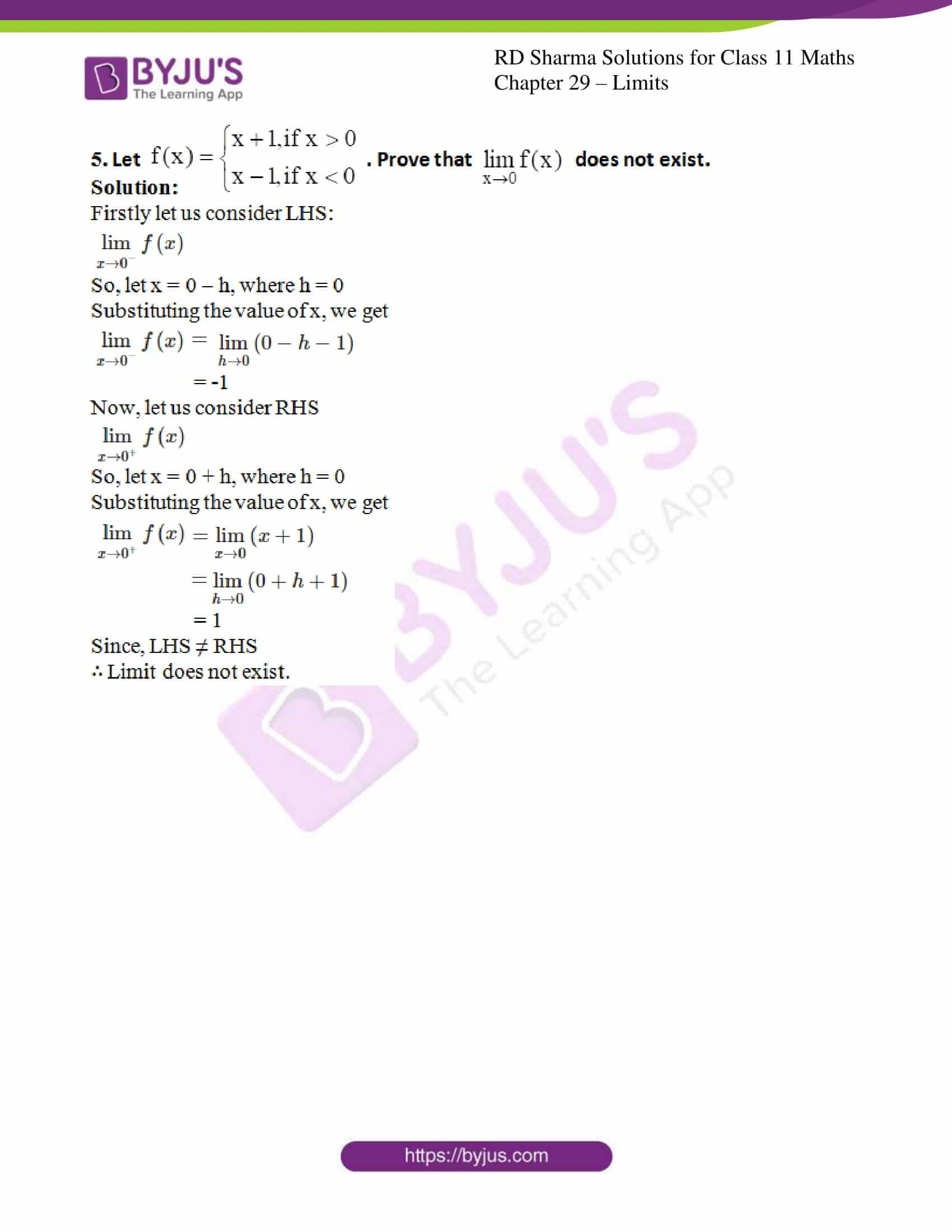### Access answers to RD Sharma Solutions for Class 11 Maths Exercise 29.1 Chapter 29 – Limits

#### EXERCISE 29.1 PAGE NO: 29.11

1. Show that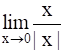does not exist.

Solution: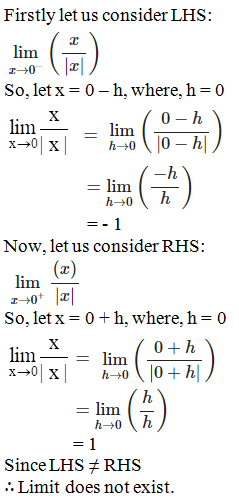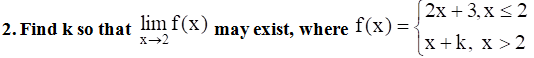Solution: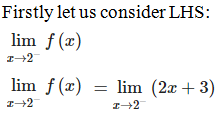So, let x = 2 – h, where h = 0

Substituting the value of x, we get